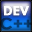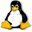Home Latest News Archive Tutorials C++ 2 3 4 Win32 Java OpenGL GLUT OpenGL ES 2 3 4 DirectX VB.NET HTML Photoshop 3dsmax Forums Projects Modelling Links About Us Search Recent Tutorials C / C++ Win32 OpenGL OpenGL ES GLUT## If Statement

Tutorials > C++ > If Statement

### Introduction

One of the main points of creating computer programs is to allow the computer to make decisions based on the current information that it has. The if statement allows you to specify exactly what will happen if a statement is true.

Contents of main.cpp :

```#include <iostream>
#include <stdlib.h>

using namespace std;

int main()
{
double bobMark = 67.5;
double fredMark = 32.3;
double sarahMark = 83.3;
```

Brackets are placed after the if statement which need to contain an expression which can evaluate to either true or false.

Please note that the action that is taken because of the if statement is part of it and therefore there is no ; after your set of expressions.

The cout statements will only run below if the conditions specified are met. Only Bob's message appears as his mark is above 50. Fred's message does not appear as his mark is below 50.

```	// Plain IF Statement
//--------------------
if (bobMark > 50)
cout << "Bob Passed" << endl;

if (fredMark > 50)
cout << "Fred Passed" << endl;
```

Another keyword available at your disposal is the else keyword. This is used in conjunction with an if. If the if statement fails, the statements in the else part will execute.

Fred's mark is less than 50 and therefore the "Fred Failed" message will be displayed.

```	// IF-ELSE statement
//-------------------
if (fredMark > 50)
cout << "Fred Passed" << endl;
else
cout << "Fred Failed" << endl;
```

Multiple if's and else's can be combined together if more than 2 possible conditions are available.

This can be shown by grouping a person into a specific category ie.

• Passed with an A
• Passed without an A
• Failed
```	// Multiple IF-ELSE's
//--------------------
if (fredMark > 80)
cout << "Fred Received an A" << endl;
else if (fredMark > 50)
cout << "Fred Passed with no A" << endl;
else
cout << "Fred Failed" << endl;
```

If you need more than 1 statement to be executed when using an if or else statement, you need to enclose your code in a code block. This is needed otherwise the computer would not have any way of deciding what should be executed and what should not.

The code below will increase Fred's mark and display a message if he has failed.

```	// IF-ELSE with code block
//-------------------------
if (fredMark < 50)
{
fredMark += 10;
cout << "We helped Fred" << endl;
}
else
cout << "Fred Passed";
```

Below is an example of a problem known as the nested if problem.

When an else statement is reached, it is recognized as being associated with the last if statement that was created.

The indentation below may trick you into believing that the else part will run if Fred's mark is not less than 50.
Because the else statement is associated with the 2nd if statement, it could be displayed if Fred's mark is less than 50 and Sarah's mark is above 50.

```	// Nested IF problem
//-------------------
if (fredMark < 50)
if (sarahMark < 50)
cout << "Fred and Sarah Both Failed"
<< endl;
else
cout << "Fred Passed"
<< endl;
```

You can solve this problem by using code blocks. If you feel that you could make this mistake often, you can place a code block whenever you have an if statement, even though only one line is being executed.

```	// Fixing the nested IF problem
//------------------------------
if (fredMark < 50)
{
if (sarahMark < 50)
cout << "Fred and Sarah Both Failed"
<< endl;
}
else
cout << "Fred Passed"
<< endl;
```

The code above basically checks if Fred's mark and Sarah's mark is less than 50. This can be combined to form a more readable statement as shown below. You can use the logical operators that you learnt in the previous tutorial to combine two statements.

```	// Multiple relational expressions
//---------------------------------
if (fredMark < 50 && sarahMark < 50)
cout << "Fred and Sarah both failed"
<< endl;
else if (fredMark > 50 && sarahMark > 50)
cout << "Fred and Sarah both passed"
<< endl;

system("pause");

return 0;
}```

Congratulations. You should now be able to utilize the if statement to its full potential.

Please let me know of any comments you may have : Contact Me

 Source Files :< Tutorial 12 - Relational Operators Tutorial 14 - Switch Statement >